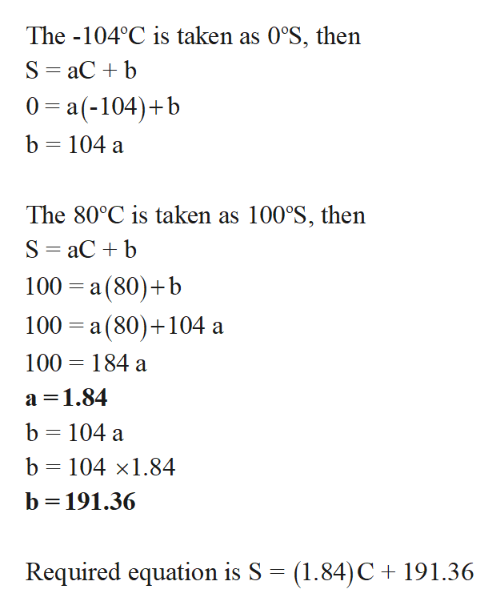Suppose that a new temperature scale has been devised on which the melting point of some compound (−104.0°C) and the boiling point of the same compound (80.0°C) are taken as 0°S and 100°S, respectively, where S is the symbol for the new temperature scale. Derive an equation relating a reading on this scale to a reading on the Celsius scale. What would this thermometer read at 32.4°C?

Question

Suppose that a new temperature scale has been devised on which the melting point of some compound (−104.0°C) and the boiling point of the same compound (80.0°C) are taken as 0°S and 100°S, respectively, where S is the symbol for the new temperature scale. Derive an equation relating a reading on this scale to a reading on the Celsius scale. What would this thermometer read at 32.4°C?

Step 1

Consider the equation S = aC+b here, S denotes new temperatu...help_outlineImage TranscriptioncloseThe -104°C is taken as 0°S, then S aCb 0 a(-104)b b 104 a The 80°C is taken as 100°S, then S aCb 100 a (80) b 100 a (80) 104 a 100 184 a a 1.84 b 104 a b 104 x1.84 b 191.36 Required equation is S (1.84)C 191.36 fullscreen

Want to see the full answer?

See Solution

Want to see this answer and more?

Our solutions are written by experts, many with advanced degrees, and available 24/7

See Solution
Tagged in

General Chemistry